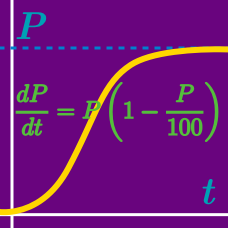Calculus

# Differential Equations - Degree and Order

Which of the following differential equations is a linear equation of order $3:$ \begin{aligned} &(a) \frac{d^3y}{dx^3}+\frac{d^2y}{dx^2}\frac{dy}{dx}+y=5x\\ &(b) \frac{d^3y}{dx^3}+\frac{d^2y}{dx^2}+y^2=x^2+3x\\ &(c) x\frac{d^3y}{dx^3}+\frac{d^2y}{dx^2}=e^x\\ &(d) \frac{d^2y}{dx^2}+\frac{dy}{dx}=\log{x}? \end{aligned}

If $a$ and $b$ are the order and the degree, respectively, of the differential equation $\left(\frac{d^{4}y}{dx^{4}}\right)^{8}-\left(\frac{dy}{dx}\right)^{25}+15=0,$ what is the value of $b-a?$

What is the degree of the differential equation $\frac{d^3y}{dx^3}+12\left(\frac{d^2y}{dx^2}\right)^2=x^3\log\frac{d^2y}{dx^2} ?$

What is the degree of the following ordinary differential equation: $k(y'')^{5}=(1+(y'')^{4})^{4}?$

Which of the following differential equations has degree $1 ?$ \begin{aligned} &\text{(a) } x^3\frac{d^2y}{dx^2}+(x+x^2)\left(\frac{dy}{dx}\right)^2+e^xy^3=2\sin x \\ &\text{(b) } y=2\frac{dy}{dx}+5\sqrt{1-\left(\frac{dy}{dx}\right)^2} \\ &\text{(c) } \sqrt{\frac{dy}{dx}+y}=x+4 \end{aligned}

×